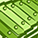# QlikView App Dev

Discussion Board for collaboration related to QlikView App Development.

Announcements
cancel
Showing results for
Did you mean:Creator II

## PIVOT TABLE TOTAL AND SUBTOTAL COLORS

Hi,

In the Pivot Table on Background Color i have given this Conditions to change the background color of Cells

=if(ID<=5 and CFS>=1,RGB(255,255,153), if(ID>=6 and ID<=10,RGB(255,204,153), If(ID>=11 and ID<=20,RGB(204,0,0) , If(ID>=21 and ID<=30,RGB(153,204,255),If(ID>=31,RGB(255,0,0))))))

But these colors are applied to Total and Grand Total Columns,But the requirement is the Total and Grandtotal columns look as it is they are in White.Can any one help me.

1 Solution

Accepted SolutionsMVP

Use dimensionality and SecondaryDimensionality to restrict the colors to only the main chart. I don't know how many dimensions you have and how many of them are pivoted. You can create an expression to check them.

dimensionality()

SecondaryDimensionality()

Let say you get 1 and 1 for them in the main chart area, then try this:

If(dimensionality() = 1 and SecondaryDimensionality() = 1, if(ID<=5 and CFS>=1,RGB(255,255,153), if(ID>=6 and ID<=10,RGB(255,204,153), If(ID>=11 and ID<=20,RGB(204,0,0) , If(ID>=21 and ID<=30,RGB(153,204,255),If(ID>=31,RGB(255,0,0)))))))

3 RepliesMVP

Use dimensionality and SecondaryDimensionality to restrict the colors to only the main chart. I don't know how many dimensions you have and how many of them are pivoted. You can create an expression to check them.

dimensionality()

SecondaryDimensionality()

Let say you get 1 and 1 for them in the main chart area, then try this:

If(dimensionality() = 1 and SecondaryDimensionality() = 1, if(ID<=5 and CFS>=1,RGB(255,255,153), if(ID>=6 and ID<=10,RGB(255,204,153), If(ID>=11 and ID<=20,RGB(204,0,0) , If(ID>=21 and ID<=30,RGB(153,204,255),If(ID>=31,RGB(255,0,0)))))))Contributor III

Hi Bhavvi,

In you "Background Color" condition you should include an IF condition to check what column number you are currently checking.

The "Total" column, should always be ColumnNo() = 0.

So if you put something like this:

```IF(ColumnNo() <> 0,
```

This will apply your conditions to all cells except the "Total" column.

Here you can see an example I ran in Qlikview:So as long as your background condition only applies for those cells that have a "ColumnNo()" different than 0, you will be coloring all cells except the total column.

Hope this is what you were looking for!

Regards!Creator II
Author

Thank you sunny.It's working